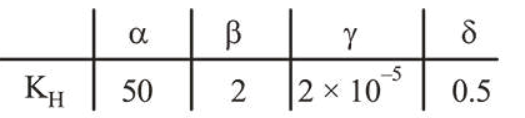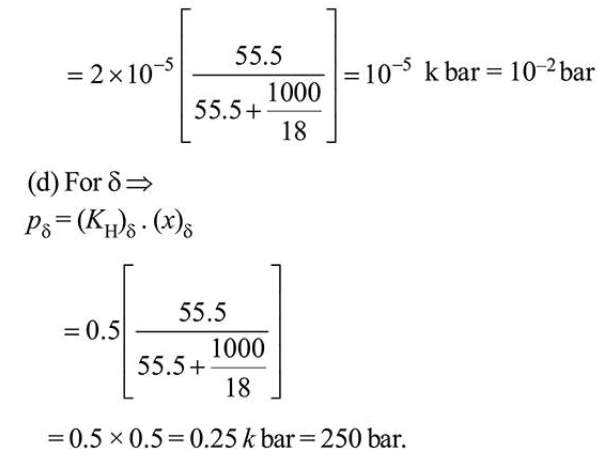# Henry's constant (in kbar) for four gasesQuestion:

Henry's constant (in kbar) for four gases $\alpha, \beta, \gamma$ and $\delta$ in water at $298 \mathrm{~K}$ is given below :(density of water $=10^{3} \mathrm{~kg} \mathrm{~m}^{-3}$ at $298 \mathrm{~K}$ )

This table implies that :

1. $\alpha$ has the highest solubility in water at a given pressure

2. solubility of $\gamma$ at $308 \mathrm{~K}$ is lower than at $298 \mathrm{~K}$

3. The pressure of a $55.5 \mathrm{molal}$ solution of $\gamma$ is $1 \mathrm{bar}$

4. The pressure of a $55.5$ molal solution of $\delta$ is $250 \mathrm{bar}$

Correct Option: , 4

Solution:

(a) From Henry's law $p=K_{\mathrm{H}}(x)$

Higher the value of $K_{\mathrm{H}}$ smaller will be the solubility of the gas, so $\gamma$ is more soluble.

(b) Though solubility of gases will decrease with increase in temperature but this conclusion can not be drawn from the given table.

(c) For $\gamma$

$(p)_{\gamma}=\left(K_{\mathrm{H}}\right)_{\gamma} \cdot(x)_{\gamma}$